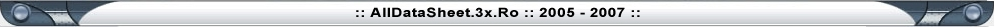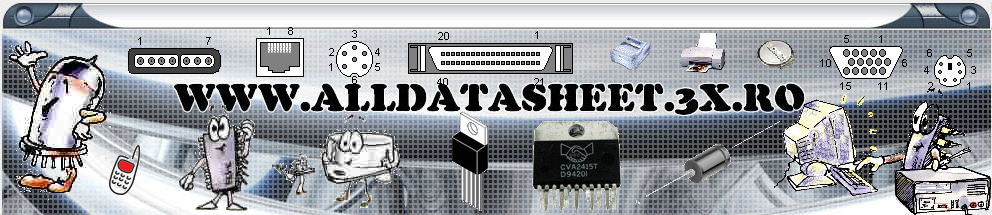Home

Capacitors

Capacitors are common components of electronic circuits, used almost as frequently as resistors. Basic difference between the two is the fact that capacitor resistance (called reactance) depends on voltage frequency, not only on capacitors' features. Common mark for reactance is Xc and it can be calculated using the following formula: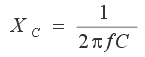f representing the frequency in Hz and C representing the capacity in Farads.

For example, 5nF-capacitor's reactance at f=125kHz equals: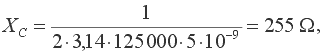while, at f=1.25MHz, it equals: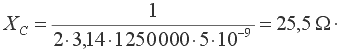Capacitor has infinitely high reactance for direct current, because f=0.

Capacitors are used in circuits for filtering signals of specified frequency. They are common components of electrical filters, oscillator circuits, etc.

Basic characteristic of capacitor is its capacity -  higher the capacity is, higher is the amount of electricity capacitor can accumulate. Capacity is measured in Farads (F). As one Farad represents fairly high capacity value, microfarad (µF), nanofarad (nF) and picofarad (pF) are commonly used. As a reminder, relations between units are:

1F=106µF=109nF=1012pF,

that is 1µF=1000nF and 1nF=1000pF. It is essential to remember this notation, as same values may be marked differently in different electrical schemes. For example, 1500pF may be used interchangeably with 1.5nF, 100nF may replace 0.1µF, etc. Bear in mind that simpler notation system is used, as with resistors. If the mark by the capacitor in the scheme reads 120 (or 120E) capacity equals 120pF, 1n2 stands for 1.2nF, n22 stands for 0.22nF, while .1µ (or .1u) stands for 0.1µF capacity and so forth.

Capacitors come in various shapes and sizes, depending on their capacity, working voltage, insulator type, temperature coefficient and other factors. All capacitors can divided in two groups: those with changeable capacity values and those with fixed capacity values. These will covered in the following chapters.

1. Block-capacitors

Capacitors with fixed capacity values (the so called block-capacitors) consist of two thin metal bands, separated by thin insulator foil. Most commonly used material for these bands is aluminum, while the common materials used for insulator foil include paper, ceramics, mica, etc after which the capacitors get named. A number of different block-capacitors are shown in the photo below. A symbol for a capacitor is in the upper right corner of the image.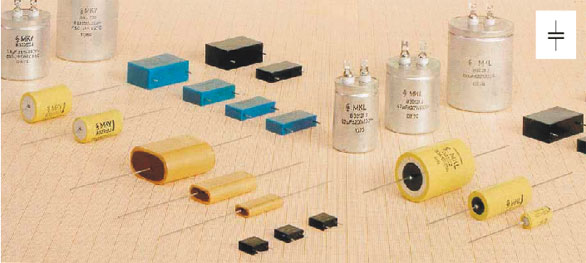Fig. 2.1: Block capacitors

Most of the capacitors, block-capacitors included, are nonpolarized components, meaning that both of their connectors are equivalent in respect of solder. Electrolytic capacitors represent the exception as their polarity is of importance, which will be covered in the following chapters.

2. Marking the block-capacitors

Commonly, capacitors are marked by a number representing the capacity value printed on the capacitor. Beside this value, number representing the maximal capacitor working voltage is mandatory, and sometimes tolerance, temperature coefficient and some other values are printed too. If, for example, capacitor mark in the scheme reads 5nF/40V, it means that capacitor with 5nF capacity value is used and that its maximal working voltage is 40v. Any other 5nF capacitor with higher maximal working voltage can be used instead, but they are as a rule larger and more expensive.

Sometimes, especially with capacitors of low capacity values, capacity may be represented with colors, similar to four-ring system used for resistors (figure 2.2). The first two colors (A and B) represent the first two digits, third color (C) is the multiplier, fourth color (D) is the tolerance, and the fifth color (E) is the working voltage.

With disk-ceramic capacitors (figure 2.2b) and tubular capacitors (figure 2.2c) working voltage is not specified, because these are used in circuits with low or no DC voltage. If tubular capacitor does have five color rings on it, then the first color represents the temperature coefficient, while the other four specify its capacity value in the previously described way.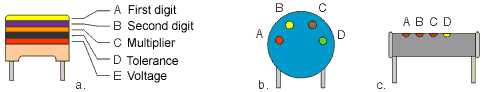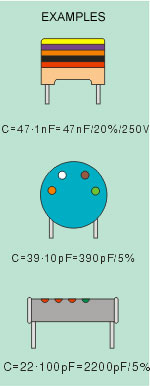COLOR DIGIT MULTIPLIER TOLERANCE VOLTAGE Black 0 x 1 pF ±20% Brown 1 x 10 pF ±1% Red 2 x 100 pF ±2% 250V Orange 3 x 1 nF ±2.5% Yellow 4 x 10 nF 400V Green 5 x 100 nF ±5% Blue 6 x 1 µF Violet 7 x 10 µF Grey 8 x 100 µF White 9 x 1000 µF ±10%

Fig. 2.2: Marking the capacity using colors

The figure 2.3 shows how capacity of miniature tantalum electrolytic capacitors is marked by colors. The first two colors represent the first two digits and have the same values as with resistors. The third color represents the multiplier, which the first two digits should be multiplied by, to get the capacity value expressed in µF. The fourth color represents the maximal working voltage value.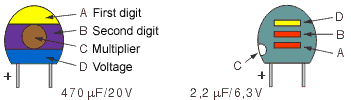COLOR DIGIT MULTIPLIER VOLTAGE Black 0 x 1 µF 10V Brown 1 x 10 µF Red 2 x 100 µF Orange 3 Yellow 4 6.3V Green 5 16V Blue 6 20V Violet 7 Grey 8 x .01 µF 25V White 9 x .1 µF 3V Pink 35V

Fig. 2.3: Marking the tantalum electrolytic capacitors

One important note on the working voltage: capacitor voltage mustn't exceed the maximal working voltage as capacitor may get destroyed. In case when the voltage between nodes where the capacitor is about to be connected is unknown, the "worst" case should be considered. There is the possibility that, due to malfunction of some other component, voltage on capacitor equals the power supply voltage. If, for example, the power supply is 12V battery, then the maximal working voltage of used capacitors should exceed 12V, for security's sake.

3. Electrolytic capacitors

Electrolytic capacitors represent the special type of capacitors with fixed capacity value. Thanks to the special construction, they can have exceptionally high capacity, ranging from one to several thousand µF. They are most frequently used in transformers for leveling the voltage, in various filters, etc.

Electrolytic capacitors are polarized components, meaning that they have positive and negative connector, which is of outmost importance when connecting the capacitor into a circuit. Positive connector has to be connected to the node with a high voltage than the node for connecting the negative connector. If done otherwise, electrolytic capacitor could be permanently damaged due to electrolysis and eventually destroyed.

Explosion may also occur if capacitor is connected to voltage that exceeds its working voltage. In order to prevent such instances, one of the capacitor's connectors is very clearly marked with a + or -, while working voltage is printed on capacitor body.

Several models of electrolytic capacitors, as well as their symbols, are shown on the picture below.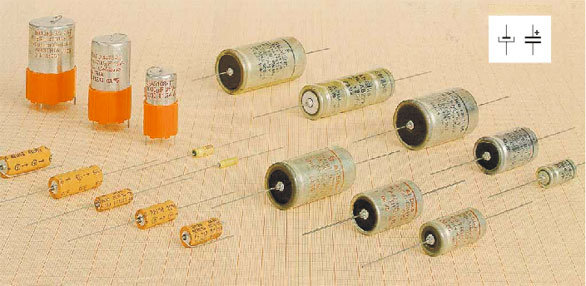Fig. 2.4: Electrolytic capacitors

Tantalum capacitors represent a special type of electrolytic capacitors. Their parasitic inductance is much lower then with standard aluminum electrolytic capacitors so that tantalum capacitor with significantly (even ten times) lower capacity can completely substitute an aluminum electrolytic capacitor.

4. Variable capacitors

Variable capacitors are capacitors with variable capacity. Their minimal capacity ranges from 10 to 50pF, and their maximum capacity goes as high as few hundred pF (500pF tops). Variable capacitors are manufactured in various shapes and sizes, but common feature for all of them is a set of immobile, interconnected aluminum plates called stator, and another set of plates, connected to a common axis, called rotor. In axis rotating, rotor plates get in between stator plates, thus increasing capacity of the device. Naturally, these capacitors are constructed in such a way that rotor and stator plates are placed consecutively. Insulator (dielectric) between the plates is a thin layer of air, hence the name variable capacitor with air dielectric. When setting these capacitors, special attention should be paid not to band metal plates, in order to prevent short-circuiting of rotor and stator and ruining the capacitor.

Bellow is the photo of the variable capacitor with air dielectric (2.5a).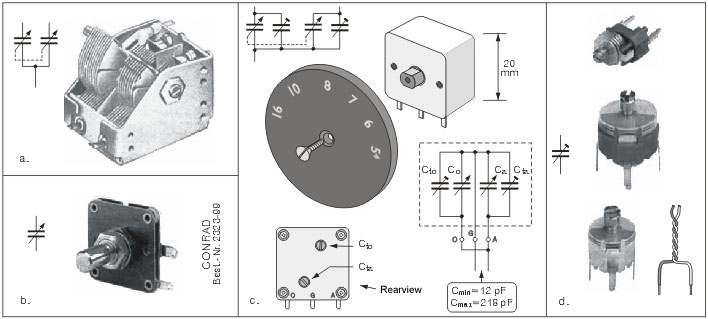Fig. 2.5: a, b, c. Variable capacitors, d. Trimmer capacitors

These are actually two capacitors with air dielectric whose rotors share the common axis, so that axis rotation changes the capacities of both capacitors. These two-fold capacitors are used in radio receivers: larger one is used in the input circuit, and the smaller one in the local oscillator. Symbol for such capacitors is shown by the photo. Contour line points to the fact that the rotors are mechanically and electrically interconnected. If one part of variable capacitor should be connected to the mass, which is often the case, then it is rotor(s).

Beside the capacitors with air dielectric, there are also variable capacitors with solid insulator. With these, thin insulator foil occupies the space between stator and rotor, while capacitor itself is contained in a plastic casing. These capacitors are much more resistant to mechanical damage and quakes, which makes them very convenient for portable electronic devices. One such one-fold capacitor is shown on the figure 2.5b.

Variable capacitors are not readily available in amateur conditions, but can be obtained from worn out radio receivers, for example (these capacitors are usually Japanese in origin). One such capacitor, used in portable radio receivers with AM area only, is shown on the figure 2.5c. The plastic casing contains four capacitors, two variacs and two trimmers, connected according to the scheme from the upper left corner. Connecting the pins according to the lower scheme gets us a one fold variable capacitor with capacity ranging from 12pF to 218pF.

The most common devices containing variable capacitors are the radio receivers, where these are used for frequency tuning. Semi-variable or trimmer capacitors are miniature capacitors, with capacity ranging from several pF to several tens of pFs. These are used for fine tuning in the radio receivers, radio transmitters, oscillators, etc. Three trimmers, along with their symbol, are shown on the figure 2.5d.

5. Practical examples with capacitors

Several practical examples with capacitors are shown on the figure 2.6. The figure 2.6a shows a 5µF electrolytic capacitor used for the signal filtering. It is used for getting the LF signal from the previous block to the transistor basis, amplifying it and reproducing via headphones. The capacitor prevents the DC from the previous block getting to the transistor basis. This occurs because the capacitor of sufficiently high capacity acts like a resistor of very low resistance for LF signals, and as a resistor of infinitely high resistance value for DC.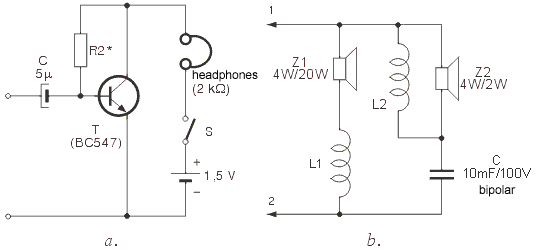Fig. 2.6: a. Amplifier with headphones, b. Electrical band-switch

The figure 2.6b represents a scheme of electrical band-switch with two speakers, with Z1 used for reproducing low and mid-frequency tones, and Z2 used for high frequency tones. Nodes 1 and 2 are connected to the audio amplifier output. Coils L1 and L2 and the capacitor C ensure that low and mid-frequency currents flow to the speaker Z1, while high frequency currents flow to Z2. How this works exactly ? In case of high frequency current, it can flow through either  Z1 and L1 or Z2 and C. Since the frequency is high, reactance (resistance) values of coils are high, while the capacitor's reactance value is low. It is clear that in this case, current will flow through Z2. In similar fashion, in case of low-frequency impulses, currents will flow through Z1, due to high capacitor reactance and low coil reactance.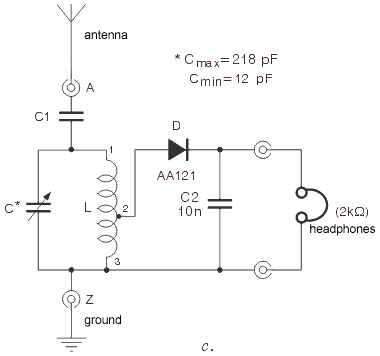Web Counter by TrafficFile.com